# 简单聊聊目标检测新范式RT-DETR的骨干：HGNetv2

【前言】前几天疯狂刷屏的RT-DETR赚足了眼球，在精度和速度上体现的优势和性价比远远高于YOLO，而今年ChatGPT、Sam的出现，也让一些吃瓜群众知乎CNN没有未来了，今天的文章，我们简单聊一聊RT-DETR的骨干网络，HGNetv2。

### 一、RT-DETR横空出世¶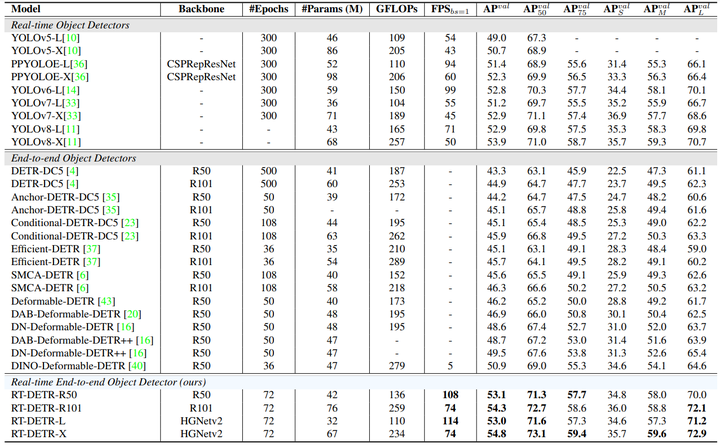### 二、初识HGNet¶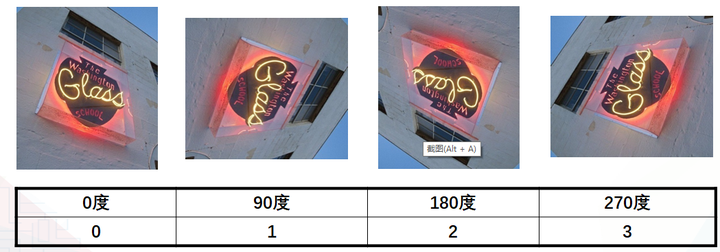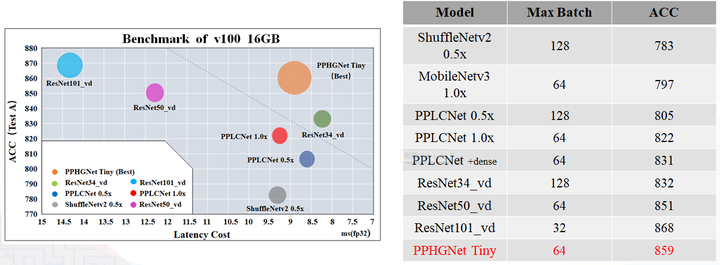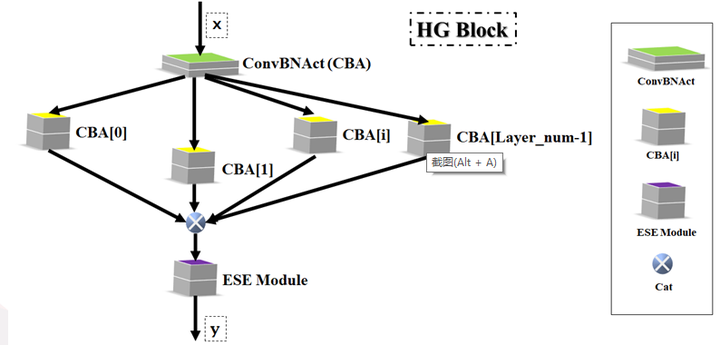ConvBNAct是啥？简单聊一聊，就是Conv+BN+Act，CV Man应该最熟悉不过了：

class ConvBNAct(TheseusLayer):
def __init__(self,
in_channels,
out_channels,
kernel_size,
stride,
groups=1,
use_act=True):
super().__init__()
self.use_act = use_act
self.conv = Conv2D(
in_channels,
out_channels,
kernel_size,
stride,
groups=groups,
bias_attr=False)
self.bn = BatchNorm2D(
out_channels,
weight_attr=ParamAttr(regularizer=L2Decay(0.0)),
bias_attr=ParamAttr(regularizer=L2Decay(0.0)))
if self.use_act:
self.act = ReLU()

def forward(self, x):
x = self.conv(x)
x = self.bn(x)
if self.use_act:
x = self.act(x)
return x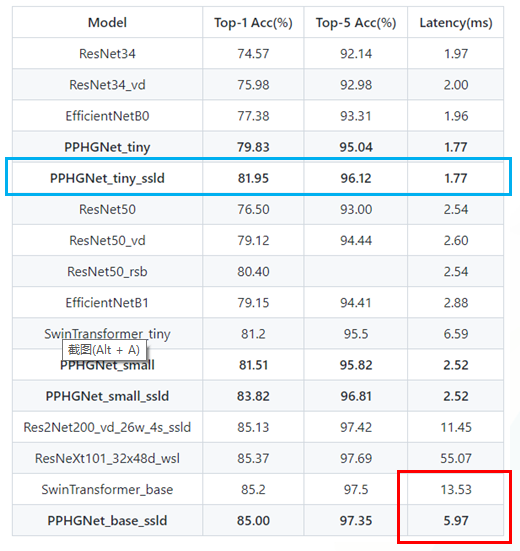• PP-HGNet 的第一层由channel为96的Stem模块构成，目的是为了减少参数量和计算量。 PP-HGNet
• Tiny的整体结构由四个HG Stage构成，而每个HG Stage主要由包含大量标准卷积的HG Block构成。
• PP-HGNet的第三到第五层使用了使用了可学习的下采样层（LDS Layer），该层group为输入通道数，可达到降参降计算量的作用，且Tiny模型仅包含三个LDS Layer，并不会对GPU的利用率造成较大影响.
• PP-HGNet的激活函数为Relu，常数级操作可保证该模型在硬件上的推理速度。

### 三、再探HGNetv2¶

# refer to https://github.com/PaddlePaddle/PaddleDetection/blob/develop/ppdet/modeling/backbones/hgnet_v2.py
class ConvBNAct(nn.Layer):
def __init__(self,
in_channels,
out_channels,
kernel_size=3,
stride=1,
groups=1,
use_act=True,
use_lab=False,
lr_mult=1.0):
super().__init__()
self.use_act = use_act
self.use_lab = use_lab
self.conv = Conv2D(
in_channels,
out_channels,
kernel_size,
stride,
if isinstance(padding, str) else (kernel_size - 1) // 2,
groups=groups,
bias_attr=False)
self.bn = BatchNorm2D(
out_channels,
weight_attr=ParamAttr(
regularizer=L2Decay(0.0), learning_rate=lr_mult),
bias_attr=ParamAttr(
regularizer=L2Decay(0.0), learning_rate=lr_mult))
if self.use_act:
self.act = ReLU()
if self.use_lab:
self.lab = LearnableAffineBlock(lr_mult=lr_mult)
# 激活函数部分添加lab结构


# refer to https://github.com/PaddlePaddle/PaddleDetection/blob/develop/ppdet/modeling/backbones/hgnet_v2.py
class LearnableAffineBlock(nn.Layer):
def __init__(self,
scale_value=1.0,
# scale设置分流占比
bias_value=0.0,
lr_mult=1.0,
lab_lr=0.01):
super().__init__()
self.scale = self.create_parameter(
shape=[1, ],
default_initializer=Constant(value=scale_value),
attr=ParamAttr(learning_rate=lr_mult * lab_lr))
self.bias = self.create_parameter(
shape=[1, ],
default_initializer=Constant(value=bias_value),
attr=ParamAttr(learning_rate=lr_mult * lab_lr))

def forward(self, x):
return self.scale * x + self.bias


PP-HGNetv2的整体结构详见下图：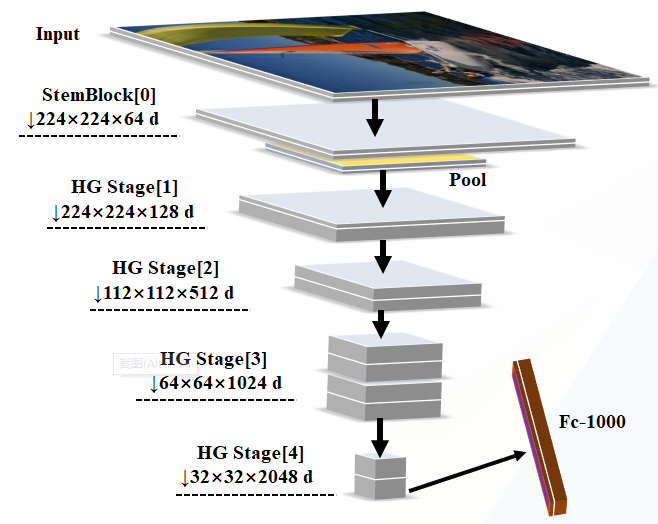【结尾】 总体而言，HGNet还是一个比较低调的网络，官方也没有过多宣传，但是好不好用，依旧还是使用者说了算，后续如果DETR变体可以在国内常见的板端成熟落地，如瑞芯微，地平线，高通等芯片上适配，会给使用者带来更多的选择。